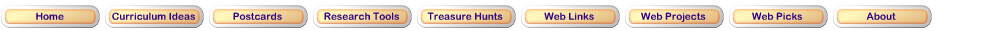Data Analysis and Probability Standard for Grades 3–5 Formulate questions that can be addressed with data and collect, organize, and display relevant data to answer them • design investigations to address a question and consider how data-collection methods affect the nature of the data set; • collect data using observations, surveys, and experiments; • represent data using tables and graphs such as line plots, bar graphs, and line graphs; • recognize the differences in representing categorical and numerical data. Select and use appropriate statistical methods to analyze data • describe the shape and important features of a set of data and compare related data sets, with an emphasis on how the data are distributed; • use measures of center, focusing on the median, and understand what each does and does not indicate about the data set; • compare different representations of the same data and evaluate how well each representation shows important aspects of the data. Develop and evaluate inferences and predictions that are based on data • propose and justify conclusions and predictions that are based on data and design studies to further investigate the conclusions or predictions. Step 1Pose this question? How many of each color are in a bag of candy such as Skittles or M&Ms? Have students make predictions about the number of each color.Step 2Download the spreadsheet for this activity. Divide into groups for this investigation. Each group will have a different kind of candy. Gather the information by sorting and counting three bags of the same candy. Type the numbers into the graph.Step 3How accurate were your predictions? What did you observe from the data? What more would you like to know?Visit these Websites to practice graphing.E-Lab Collect and organize data.E-Lab Make a bar graph.E-Lab Record the results of experiments.E-Lab Find the median and mode in this activityE-Lab Predict outcomes in this activityKidsZone Make an area, pie, line, bar, or xy graph with this interactive tool.Scholastic Study Jams Play games to understand data and graphing vocabulary.Updated March 14, 2017
© 1996 - 2015 Linda C. Joseph